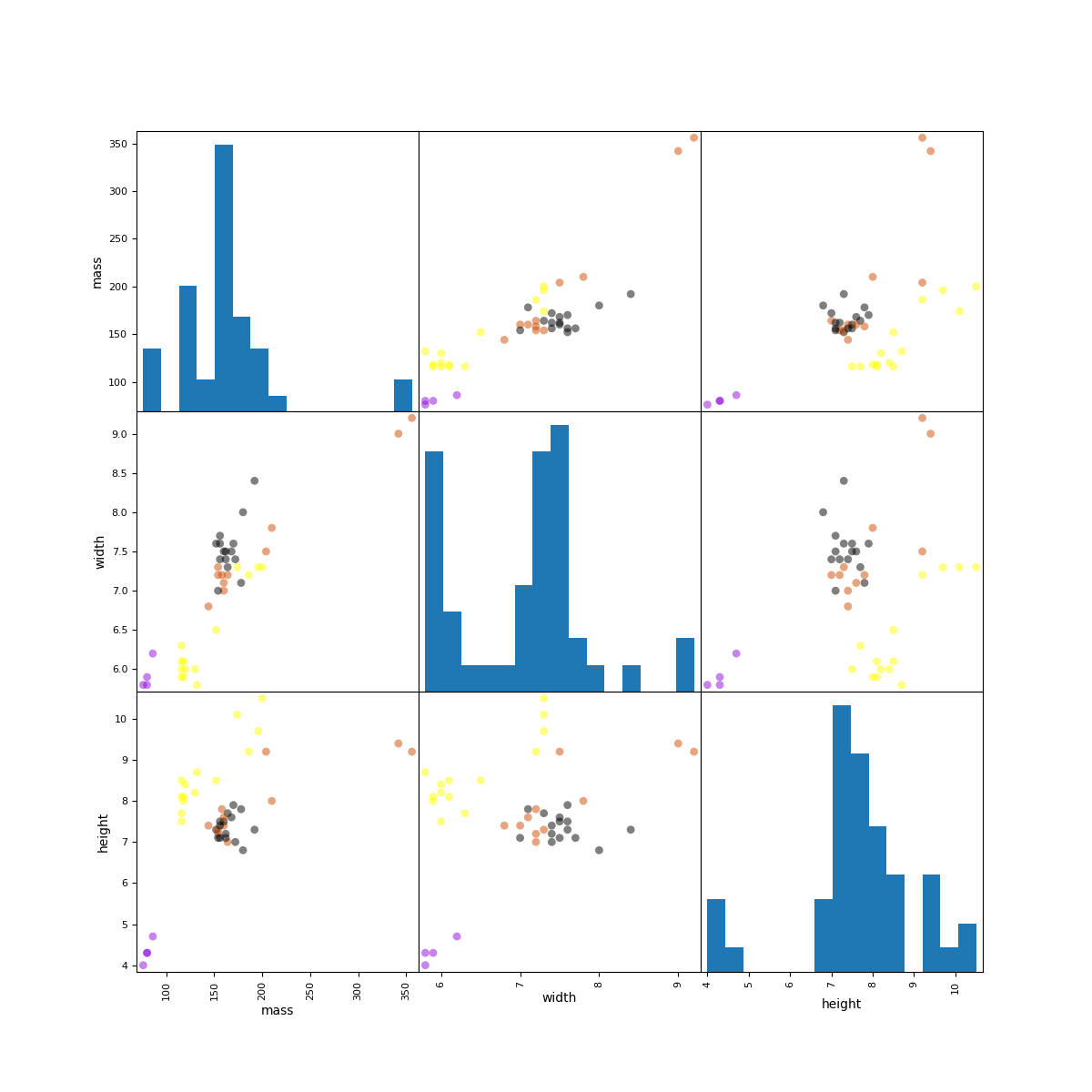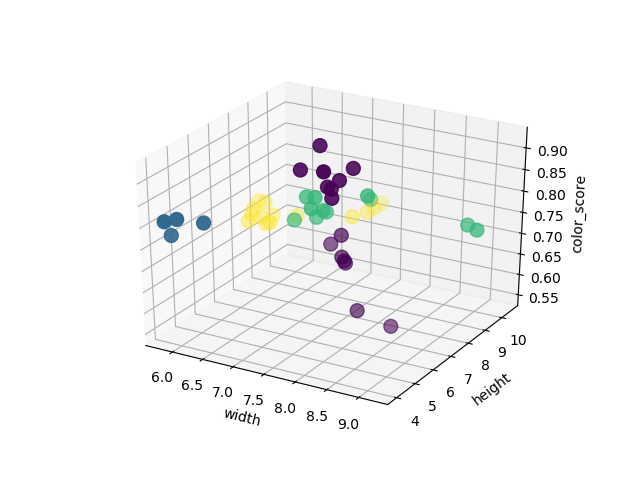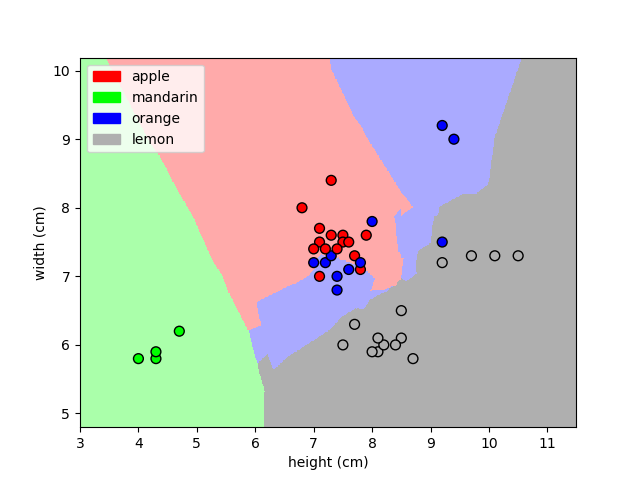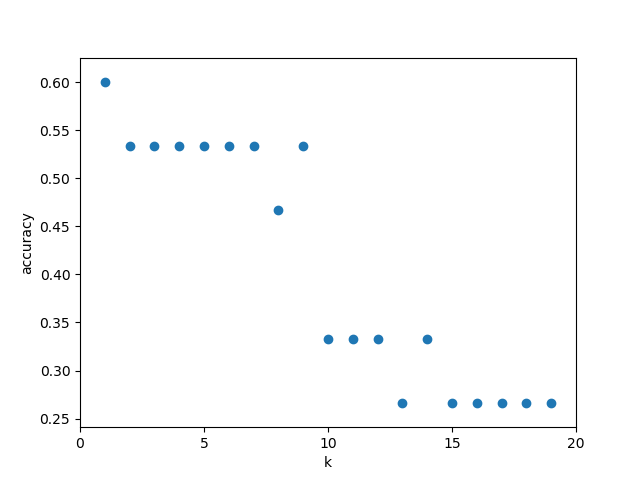# Module 1: Fundamentals of Machie Learning - Intro to Scikit Learn¶

### Kevyn Collins-Thompson¶

Associate Professor of Information and Computer Science

In :
%matplotlib notebook
import numpy as np
import matplotlib.pyplot as plt
import pandas as pd
from sklearn.model_selection import train_test_split

In :
fruits = pd.read_table('./fruit_data_with_colors.txt')

In :
fruits.head()

Out:
fruit_label fruit_name fruit_subtype mass width height color_score
0 1 apple granny_smith 192 8.4 7.3 0.55
1 1 apple granny_smith 180 8.0 6.8 0.59
2 1 apple granny_smith 176 7.4 7.2 0.60
3 2 mandarin mandarin 86 6.2 4.7 0.80
4 2 mandarin mandarin 84 6.0 4.6 0.79
In :
fruits.shape

Out:
(59, 7)
In :
lookup_fruit_name = dict(zip(fruits.fruit_label.unique(), fruits.fruit_name.unique()))
lookup_fruit_name

Out:
{1: 'apple', 2: 'mandarin', 3: 'orange', 4: 'lemon'}

# Create train-test split¶

In :
X = fruits[['mass', 'width', 'height','color_score']]
y = fruits['fruit_label']

#In this example. we're using a 75%-25% split of training versus test data
#It's a good rule of thumb to use in deciding what proportion of training
#versus test might be helpful
X_train, X_test, y_train, y_test = train_test_split(X, y, random_state = 0)


We'll denote the data that we have using different flavors of the variable X, capital X, which is typically a two dimensional array or data frame, and the notation we'll use for labels will be typically based on lowercaes y, which is usually a one dimensional array, or a scalar.

Note the use of the random_state parameter in the train_test_split() function. This random_state parameter provides a seed value to the function's internal random number generator. If we choose different values for that seed value, that will result in different randomized splits for training and test.

In :
X_train.shape

Out:
(44, 4)
In :
X_test.shape

Out:
(15, 4)
In :
y_train.shape

Out:
(44,)
In :
y_test.shape

Out:
(15,)
In :
X_train

Out:
mass width height color_score
42 154 7.2 7.2 0.82
48 174 7.3 10.1 0.72
7 76 5.8 4.0 0.81
14 152 7.6 7.3 0.69
32 164 7.2 7.0 0.80
49 132 5.8 8.7 0.73
29 160 7.0 7.4 0.81
37 154 7.3 7.3 0.79
56 116 5.9 8.1 0.73
18 162 7.5 7.1 0.83
55 116 6.3 7.7 0.72
27 204 7.5 9.2 0.77
15 156 7.7 7.1 0.69
5 80 5.8 4.3 0.77
31 210 7.8 8.0 0.82
16 156 7.6 7.5 0.67
50 130 6.0 8.2 0.71
20 160 7.5 7.5 0.86
51 116 6.0 7.5 0.72
8 178 7.1 7.8 0.92
13 164 7.3 7.7 0.70
25 356 9.2 9.2 0.75
17 168 7.5 7.6 0.73
58 118 6.1 8.1 0.70
57 152 6.5 8.5 0.72
52 118 5.9 8.0 0.72
38 158 7.2 7.8 0.77
1 180 8.0 6.8 0.59
12 154 7.0 7.1 0.88
45 186 7.2 9.2 0.72
24 342 9.0 9.4 0.75
6 80 5.9 4.3 0.81
23 170 7.6 7.9 0.88
36 160 7.1 7.6 0.76
21 156 7.4 7.4 0.84
19 162 7.4 7.2 0.85
9 172 7.4 7.0 0.89
39 144 6.8 7.4 0.75
54 116 6.1 8.5 0.71
3 86 6.2 4.7 0.80
0 192 8.4 7.3 0.55
53 120 6.0 8.4 0.74
47 196 7.3 9.7 0.72
44 200 7.3 10.5 0.72
In :
y_train

Out:
42    3
48    4
7     2
14    1
32    3
49    4
29    3
37    3
56    4
18    1
55    4
27    3
15    1
5     2
31    3
16    1
50    4
20    1
51    4
8     1
13    1
25    3
17    1
58    4
57    4
52    4
38    3
1     1
12    1
45    4
24    3
6     2
23    1
36    3
21    1
19    1
9     1
39    3
54    4
3     2
0     1
53    4
47    4
44    4
Name: fruit_label, dtype: int64
In :
X_test

Out:
mass width height color_score
26 362 9.6 9.2 0.74
35 150 7.1 7.9 0.75
43 194 7.2 10.3 0.70
28 140 6.7 7.1 0.72
11 172 7.1 7.6 0.92
2 176 7.4 7.2 0.60
34 142 7.6 7.8 0.75
46 216 7.3 10.2 0.71
40 154 7.1 7.5 0.78
22 140 7.3 7.1 0.87
4 84 6.0 4.6 0.79
10 166 6.9 7.3 0.93
30 158 7.1 7.5 0.79
41 180 7.6 8.2 0.79
33 190 7.5 8.1 0.74
In :
y_test

Out:
26    3
35    3
43    4
28    3
11    1
2     1
34    3
46    4
40    3
22    1
4     2
10    1
30    3
41    3
33    3
Name: fruit_label, dtype: int64

# Examining the Data¶

In general, if you're thinking about applying machine learning to a data set, it's a really good first step to actually look at the data set first, maybe using some simple visualization methods, or even simply scrolling through to look at the various values in the different rows before proceeding.

## Some reasons why looking at the data initially is important¶

• Inspecting feature values may help identify what cleaning or preprocessing still needs to be done once you can see the range or distribution of values that is typical for each attribute.
• You might notice missing or noisy data, or inconsistencies such as the wrong data type being used for a column, incorrect units of measurements for a particular column, or that there aren't enough examples of a particular class.
• You may realize that your problem is actually solvable without machine learning.

So now that we have a training set selected, let's create some simple visualizations to look at how the features in the objects in the training set, in our case different fruits, relate to each other and to the labals. With these visualizations, we get at least two major benefits:

First, we can get an idea of the range of values that each feature takes on, and we can immediately see any unusual outliers that are very different from other points, and that might indicate noise or a missing feature or other problem with the data set.

Second, we may be able to get a better idea how likely it is that a machine learning algorithm could do well at predicting the different classes. By seeing how well clustered and well separated the different types of objects are in feature space. So feature space refers to the representation of an object using specific features that are in certain columns of the data that we have.

## feature pair plot¶

In :
from matplotlib import cm
cmap = cm.get_cmap('gnuplot')
scatter = pd.scatter_matrix(X_train, c= y_train, marker = 'o', s=40, hist_kwds={'bins':15}, figsize = (12,12), cmap = cmap)

/home/sabodhapati/anaconda3/lib/python3.6/site-packages/ipykernel_launcher.py:3: FutureWarning: pandas.scatter_matrix is deprecated. Use pandas.plotting.scatter_matrix instead
This is separate from the ipykernel package so we can avoid doing imports until## A three-dimensional feature scatterplot¶

In :
from mpl_toolkits.mplot3d import Axes3D
fig = plt.figure()
ax = fig.add_subplot(111, projection = '3d')
ax.scatter(X_train['width'], X_train['height'], X_train['color_score'], c = y_train, marker = 'o', s=100)
ax.set_xlabel('width')
ax.set_ylabel('height')
ax.set_zlabel('color_score')
plt.show()# K-Nearest Neighbors Classification¶

The K-Nearest Neighbors algorithm can be used for classification and regression. k-NN classifiers are an example of what's called instance based or memory based supervised learnig. What this means is that instance based learning methods work by memorizing the labeled examples that they see in the training set, and then they use those memorized examples to classify new objects later

## k-NN Classifier Algorithm¶

Given a training set X_train with labels y_train, and given a new instance x_test to be classified:

1. Find the most similar instances (let's call them X_NN) to x_test that are in X_train.

2. Get the labels y_NN for the instances in X_NN.

3. Predict the label for x_test by combining the labels y_NN, e.g. simple majority vote.

### A nearest neighbor algorithm needs four things specified:¶

1.A distance metric, e.g. a straight line or euclidean distance to measure the distance between points (Minkowski with p = 2)

2.How many 'nearest' neighbors to look at? e.g. five

3.Optional weighting function on the neighbor points, i.e. give some neighbors more influence on the outcome

4.Method for aggregating the classes of neighbor points to produce a final prediction (Class with the most representatives among nearest neighbors)

### import data¶

In :
%matplotlib notebook
import numpy as np
import matplotlib.pyplot as plt
import pandas as pd
from sklearn.model_selection import train_test_split

In :
fruits = pd.read_table('./fruit_data_with_colors.txt')

Out:
fruit_label fruit_name fruit_subtype mass width height color_score
0 1 apple granny_smith 192 8.4 7.3 0.55
1 1 apple granny_smith 180 8.0 6.8 0.59
2 1 apple granny_smith 176 7.4 7.2 0.60
3 2 mandarin mandarin 86 6.2 4.7 0.80
4 2 mandarin mandarin 84 6.0 4.6 0.79
In :
lookup_fruit_name = dict(zip(fruits.fruit_label.unique(), fruits.fruit_name.unique()))
lookup_fruit_name

Out:
{1: 'apple', 2: 'mandarin', 3: 'orange', 4: 'lemon'}

### Creat train-test split¶

In :
X = fruits[['mass', 'width', 'height']]
y = fruits['fruit_label']

X_train, X_test, y_train, y_test = train_test_split(X, y, random_state = 0)


### Creat classifier object¶

In :
from sklearn.neighbors import KNeighborsClassifier

knn = KNeighborsClassifier(n_neighbors = 5)


the k-NN classifier that are using in this case is an example of a more general class called an estimator in scikit-learn.

### Train the classifier (fit the estimator) using the training data¶

All estimators have a fit method that takes the training data, and then changes the state of the classifier or estimator object to essentially enable prediction once the training is finished. In other words, it updates the state of the k and n variable here, which means that in the case of k-NN neighbors it will memorize the training set examples in some kind of internal storage fro future use.

In :
knn.fit(X_train, y_train)

Out:
KNeighborsClassifier(algorithm='auto', leaf_size=30, metric='minkowski',
metric_params=None, n_jobs=1, n_neighbors=5, p=2,
weights='uniform')

### Estimate the accuracy of the classifier on future data, using the test data¶

The first thing we can do with this newly trained classifier is to see how accurate it's likely to be on some new, previously unseen instances. To do this, we can apply the classifier to all the instances in the test set.

One simple way to assess if the classifier is likely to be good at predicting the label of future, previously unseen data instances is to compute the classifier's accuracy on the test set data items

Remember that the k-NN classifier did not see any of the fruits in the test set during the training phase.

In :
knn.score(X_test, y_test)

Out:
0.53333333333333333

This will take the test set points as input and compute the accuracy. The accuracy is defined as the fraction of test set items, whose true label was correctly predicted by the classifier.

### Use the trained k-NN classifier model to classify new, previously unseen objects¶

In :
fruit_prediction = knn.predict([[20, 4.3, 5.5]])
lookup_fruit_name[fruit_prediction]

Out:
'mandarin'
In :
fruit_prediction = knn.predict([[100, 6.3, 8.5]])
lookup_fruit_name[fruit_prediction]

Out:
'lemon'

### Plot the decision boundaries of the k-NN classifier¶

Let's use a utility function called plot_fruit_knn that's included in adspy_shared_utilities module

In :
from adspy_shared_utilities import plot_fruit_knn
plot_fruit_knn(X_train, y_train, 5, 'uniform')The uniform parameter that pass at the last parameter is the waiting method to be used. In this case uniform means to treat all neighbors equally when combining their labels. If you like, you can try changing this to the word distance, you can also pass your own function.

We can see that when k has a small value like 1, the classifier is good at learning the classes for individual points in the training set. But with a decision boundary that's fragmented with considerable variation. This is because when k = 1, the prediction is sensitive to noise, outliers, mislabeled data, and other sources of variation in individual data points.

For larger values of k, the areas assigned to different classes are smoother and not as fragmented and more robust to noise in the individual points. But possibly with some mistakes, more mistakes in individual points. This is an example of what's known as the bias / variance tradeoff.

### How sensitive is k-NN classification accuracy to the choice of the 'k' parameter?¶

In :
k_range = range(1,20)
scores = []

for k in k_range:
knn = KNeighborsClassifier(n_neighbors = k)
knn.fit(X_train, y_train)
scores.append(knn.score(X_test, y_test))

plt.figure()
plt.xlabel('k')
plt.ylabel('accuracy')
plt.scatter(k_range, scores)
plt.xticks([0,5,10,15,20])Out:
([<matplotlib.axis.XTick at 0x7f42f6f9a4e0>,
<matplotlib.axis.XTick at 0x7f42f6f87c88>,
<matplotlib.axis.XTick at 0x7f42f6fa4eb8>,
<matplotlib.axis.XTick at 0x7f42f7697da0>,
<matplotlib.axis.XTick at 0x7f42f76a0438>],
<a list of 5 Text xticklabel objects>)

Keep in mind: these results are only for this particular training test split, to get a more reliable estimate of likely future accuracy for a particular value of k, we would want to look at results over multiple possible train test splits

In general, the best choice of the value of k, that is the one that leads to the highest accuracy, can vary greatly depending on the data set. In general with k-nearest neighbors using a larger k suppresses the effects of noisy individual labels, but results in classification boundaries that are less detailed